# JP2006114974A - Voltage-controlled piezoelectric oscillator capable of linear frequency control - Google Patents

## Info

Publication number
JP2006114974A
JP2006114974A JP2004297668A JP2004297668A JP2006114974A JP 2006114974 A JP2006114974 A JP 2006114974A JP 2004297668 A JP2004297668 A JP 2004297668A JP 2004297668 A JP2004297668 A JP 2004297668A JP 2006114974 A JP2006114974 A JP 2006114974A
Authority
JP
Japan
Prior art keywords
variable capacitance
variable
capacitance diode
diode
voltage
Prior art date
Legal status (The legal status is an assumption and is not a legal conclusion. Google has not performed a legal analysis and makes no representation as to the accuracy of the status listed.)
Withdrawn
Application number
JP2004297668A
Other languages
Japanese (ja)
Other versions
JP2006114974A5 (en
Inventor
Tomio Sato

Original Assignee
Epson Toyocom Corp
エプソントヨコム株式会社
Priority date (The priority date is an assumption and is not a legal conclusion. Google has not performed a legal analysis and makes no representation as to the accuracy of the date listed.)
Filing date
Publication date
Application filed by Epson Toyocom Corp, エプソントヨコム株式会社 filed Critical Epson Toyocom Corp
Priority to JP2004297668A priority Critical patent/JP2006114974A/en
Publication of JP2006114974A5 publication Critical patent/JP2006114974A5/ja
Publication of JP2006114974A publication Critical patent/JP2006114974A/en
Withdrawn legal-status Critical Current

• 239000003990 capacitor Substances 0.000 claims abstract description 21
• 238000003780 insertion Methods 0.000 claims 1
• 238000010586 diagrams Methods 0.000 description 9
• 238000004088 simulation Methods 0.000 description 6
• 239000011651 chromium Substances 0.000 description 3
• 230000005540 biological transmission Effects 0.000 description 1
• 230000001413 cellular Effects 0.000 description 1
• 239000002131 composite materials Substances 0.000 description 1
• 239000000203 mixtures Substances 0.000 description 1
• 230000000051 modifying Effects 0.000 description 1
• 239000010955 niobium Substances 0.000 description 1

## Images

•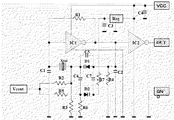•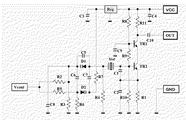•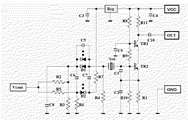•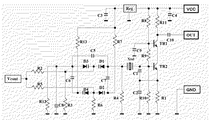•••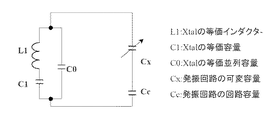•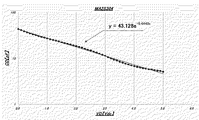•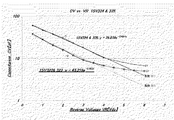•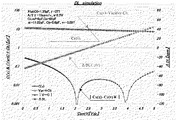•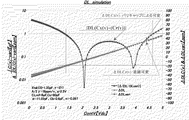•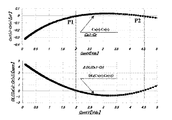•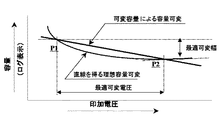•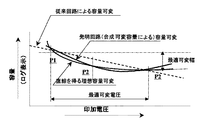•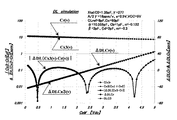•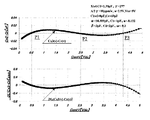## Classifications

• HELECTRICITY
• H03BASIC ELECTRONIC CIRCUITRY
• H03BGENERATION OF OSCILLATIONS, DIRECTLY OR BY FREQUENCY-CHANGING, BY CIRCUITS EMPLOYING ACTIVE ELEMENTS WHICH OPERATE IN A NON-SWITCHING MANNER; GENERATION OF NOISE BY SUCH CIRCUITS
• H03B5/00Generation of oscillations using amplifier with regenerative feedback from output to input
• H03B5/30Generation of oscillations using amplifier with regenerative feedback from output to input with frequency-determining element being electromechanical resonator
• H03B5/32Generation of oscillations using amplifier with regenerative feedback from output to input with frequency-determining element being electromechanical resonator being a piezo-electric resonator
• H03B5/36Generation of oscillations using amplifier with regenerative feedback from output to input with frequency-determining element being electromechanical resonator being a piezo-electric resonator active element in amplifier being semiconductor device
• HELECTRICITY
• H03BASIC ELECTRONIC CIRCUITRY
• H03KPULSE TECHNIQUE
• H03K3/00Circuits for generating electric pulses; Monostable, bistable or multistable circuits
• H03K3/02Generators characterised by the type of circuit or by the means used for producing pulses
• H03K3/027Generators characterised by the type of circuit or by the means used for producing pulses by the use of logic circuits, with internal or external positive feedback
• H03K3/03Astable circuits
• H03K3/0307Stabilisation of output, e.g. using crystal
• HELECTRICITY
• H03BASIC ELECTRONIC CIRCUITRY
• H03BGENERATION OF OSCILLATIONS, DIRECTLY OR BY FREQUENCY-CHANGING, BY CIRCUITS EMPLOYING ACTIVE ELEMENTS WHICH OPERATE IN A NON-SWITCHING MANNER; GENERATION OF NOISE BY SUCH CIRCUITS
• H03B2201/00Aspects of oscillators relating to varying the frequency of the oscillations
• H03B2201/02Varying the frequency of the oscillations by electronic means
• H03B2201/0208Varying the frequency of the oscillations by electronic means the means being an element with a variable capacitance, e.g. capacitance diode

## Abstract

<P>PROBLEM TO BE SOLVED: To configure a correction circuit using a variable capacity diode for performing more highly accurate compensation by configuring an approximate curve for acquiring a plurality of cross points for an ideal capacitance variation curve for acquiring a straight variation. <P>SOLUTION: As a variable capacity means forming the voltage-controlled type piezoelectric oscillator, the device includes a first variable capacitance diode, a first capacitor connected in parallel to the first variable capacitance diode, a second variable capacitance diode, a second capacitor inserted and connected between the cathode of the first variable capacity diode and an anode of the second variable capacitance diode, and a third capacitor inserted and connected between the anode of the first variable capacitance diode and the cathode of the second variable capacitance diode. The external control voltage is applied to the first variable capacitance diode and to the second variable capacitance diode so that their polarities may be opposite to each other. <P>COPYRIGHT: (C)2006,JPO&NCIPI

## Description

The present invention relates to a voltage controlled piezoelectric oscillator capable of linear frequency control.

The circuit configuration of a piezoelectric oscillator that uses a variable capacitance diode (= Variable Capacitance Diorde) to vary the oscillation frequency is, for example, the voltage control type shown in pages 17 and 18 of QIAJ's “Explanation and Application of Crystal Devices (2002.3)” As summarized in the crystal oscillator (VCXO = Voltage Controlled crystal oscillator), a variable-capacitance diode is inserted into the oscillation loop, a control voltage is applied to the variable-capacitance diode from the outside, and the capacitance change of the variable-capacitance diode causes the oscillation loop to The frequency is varied by changing the load capacity.

FIG. 5 is a circuit example of a general voltage controlled crystal oscillator disclosed in, for example, Japanese Patent Application Laid-Open No. 09-214250, and an inverter element IC1 is used for an oscillation circuit, and the input and output of the inverter IC1 are connected. A high resistance R1 is inserted into and the operating point is always set to the center. Further, between the input and output of the inverter IC1, a crystal resonator Xtal and a variable capacitance diode D1 connected in parallel with a capacitor C5 are inserted in series as variable capacitance means to form an oscillation loop. The variable range can be finely adjusted by connecting a capacitor in parallel with the variable capacitance diode. Further, capacitors C1 and C2 are inserted between the input and output of the inverter IC1 and GND, respectively.

The external control voltage Vcont is divided by the high resistors R2 and R3 and applied to the cathode of D1, the anode of D1 is grounded by the high resistor R4, and the capacitance is determined by the voltage between the cathode and anode of the variable capacitance diode D1. Since the load capacitance of the oscillation loop changes due to the change, the oscillation frequency changes. The variable width and linearity of the frequency can be finely adjusted by the element values of the high resistance R3 and the capacitor C5.

FIG. 6 shows another conventional example used for high-frequency oscillation, in which two transistors (Tr1, Tr2) are connected in cascade in the oscillation circuit. In addition, two variable capacitance diodes (D1, D2) are connected in series to prevent deterioration of noise characteristics due to modulation by the oscillation level.

Conventionally, the frequency offset at the time of capacitive load of the piezoelectric oscillation circuit is approximately given by the equation (1) as DL (= Fractional loard resonance frequency offset), and the equivalent circuit model is shown in FIG. The load capacity (= CL) of the transmission loop can be expressed as a configuration in which a circuit capacity (= Cc) and a variable capacity (= Cx) are connected in series.

In addition, by setting a reference capacity (= CLref) that gives a nominal frequency, the frequency offset amount is expressed by equation (2).

The frequency change ΔDL from the nominal frequency is the difference between Equation (1) and Equation (2), and can be expressed by Equations (3) to (4).

Examples of variable capacitance characteristics of the variable capacitance diode are shown in FIGS. From the figure, the variable characteristic of the variable capacitance diode can be approximated by equation (5), and equation (6) is obtained as variable capacitance Cx.

By substituting the equation (6) into the equation (4), the equation (7) is obtained as a relational expression indicating the variable voltage applied to the variable capacitance diode by the external control voltage and the frequency change.

Equation (8) giving the linear frequency change with respect to the variable voltage is shown.

Equation (8) is modified to give a linear frequency change with respect to the variable voltage. Equation (9) is shown.

FIG. 10 shows a simulation result example of the equations (5), (6), and (9).
Here, the crystal resonator Xtal is At-Cut, and the resonance frequency is 13 MHz. Further, C0 = 1.35 pF, γ = 277, and the circuit capacitance was Cc = 60 pF. Furthermore, the variable voltage Vcont ± 2.5 Vdc and the variable width ΔDL ± 45 ppm are set.

The variable capacitance C (v) for obtaining a straight line is a curve that is approximately 11.8 pF at 0 V and approximately 7.5 pF at 5 V.
The combined capacitance of the variable capacitance diode Vbari and the parallel capacitance Cb, which shows characteristics very close to the same variable capacitance, is shown in equation (10).

In addition, the difference between the capacitance of C (v) and the variable capacitance diode combined capacitance at that time is ≈0.3 pF or less at maximum.

FIG. 11 shows the frequency variable by the variable capacitor C (v) for obtaining a straight line and the approximate variable capacitor diode by the combined capacitor and the difference. A variable width of 70 ppm is obtained with a difference of 1 ppm. This value is considered to indicate the compensation limit by the variable capacitance diode.

The results shown in FIG. 12 show the capacitance difference between the ideal capacitance variable and the approximate variable capacitance diode, and the frequency deviation due to the capacitance difference.

An outline of the contents of FIG. 12 is shown in FIG. In other words, “ideal variable capacitance to obtain a straight line” and “variable capacitance of variable capacitance diode” according to log change intersect at two points, P1 and P2, and the best characteristics in the vicinity between the two points. It shows that it can be obtained, and also shows the limit of correction.
JP 09-214250 A Japanese Patent Laid-Open No. 10-056330

As shown in FIGS. 12 and 13, in the conventional frequency control by unidirectional voltage control to the variable capacitance diode, there is a limit to the correction amount for obtaining a straight line.
The purpose is to construct a correction circuit using variable capacitance diodes that perform more accurate compensation by constructing an approximate curve that obtains multiple intersections on the ideal capacitance change curve for obtaining linear variation. To do.

In order to solve the above problem, the present invention provides a first variable capacitance diode and a first capacitor connected in parallel with the first variable capacitance diode as variable capacitance means constituting a voltage controlled piezoelectric oscillator. The second variable capacitance diode, a second capacitor inserted and connected between the cathode of the first variable capacitance diode and the anode of the second variable capacitance diode, and the first variable capacitance diode And a third capacitor inserted and connected between the anode of the second variable capacitance diode and the cathode of the second variable capacitance diode, and the external control voltage is respectively applied to the first variable capacitance diode and the second variable capacitance diode. It is what applied so that polarity may become reverse direction, It is characterized by the above-mentioned.

In the correction by unidirectional application to the conventional variable capacitance diode, as shown in FIGS. 12 and 13, the ideal capacitance variable curve and the approximate variable capacitance diode combined capacitance variable curve have no more than two intersections even when the optimum constant is set. In contrast to this, in the present invention, as shown in FIG. 15, a second variable capacitance diode is provided, and by adding a second correction by applying a reverse voltage, it becomes possible to perform a linear correction to obtain an intersection of three points. Wide and highly accurate correction is possible.

This technology can be applied to highly accurate temperature compensated oscillators (TCXOs) and voltage controlled oscillators (VCXOs) that achieve linearity over a wider range, and communication devices that require frequency references such as mobile phones. Can greatly contribute to the improvement of performance.

Hereinafter, the present invention will be described in detail based on exemplary embodiments.
FIG. 1 is a circuit diagram showing a first embodiment of a voltage controlled piezoelectric oscillator according to the present invention. The same parts as those in the conventional circuit shown in FIG. Omitted to avoid duplication.

In the conventional circuit of FIG. 5, a capacitor C5 connected in parallel to the variable capacitance diode D1 and further connected in series to the vibrator is inserted into the oscillation loop as the variable capacitance means. The external control voltage Vcont was divided by the high resistances R2 and R3 and applied to the cathode of the variable capacitance diode D1, and the capacitance was changed by the voltage change and the oscillation frequency was controlled.
On the other hand, in the circuit of FIG. 1, as the variable capacitance means, the anode of the second variable capacitance diode D2 is connected to the cathode of the first variable capacitance diode D1 via the capacitor C6, and the second variable capacitance diode D2 The cathode is connected to the anode of the first variable capacitance diode D1 via the capacitor C7, and the cathode of the same D2 is connected to the reference power supply Reg via the high resistance R7 and pulled up.

The external control voltage Vcont is divided by the high resistances R5 and R6 and applied to the anode of the second variable capacitance diode D2 simultaneously with the conventional control via the resistor R2. As a result, the potential between the cathode and anode of the variable capacitance diode D2 decreases as the control voltage increases, and the variable capacitance of the variable capacitance diode D2 decreases.

Assuming that the combined capacitance of the variable capacitance means using only the conventional variable capacitance diode D1 and the capacitor C5 is Cx1, it can be expressed by equations (11) and (12) as in equations (5) and (6). .

If the voltage applied to the second variable capacitance diode D2 is Vari2 and is shown in Equation (13), and the combined capacitance of the series capacitance Cd is Cx2, then it is given by Equation (14). Assuming that the combined capacity of Cx1 and Cx2 is Cx3, the following equation (15) is obtained.

FIG. 2 shows a second embodiment. The variable capacitance means according to the present invention is an oscillation circuit in which two transistors most often used for reference oscillation such as a cellular phone are connected in cascade. It is applied. The configuration and operation of the variable capacitance means are basically the same as those of the circuit of FIG.

FIG. 3 shows a third embodiment, in which a plurality of variable capacitance diodes connected in parallel as the first and second variable capacitance diodes are respectively arranged and combined with the variable capacitance diodes at zero bias. The amount of change with respect to the capacity and the control voltage can be adjusted to an appropriate value. That is, by combining a plurality of variable capacitance diodes having different characteristics, an excellent variable capacitance means that cannot be obtained by a single variable capacitance diode can be realized.

FIG. 4 shows a fourth embodiment. The first and second variable capacitance diodes are arranged by connecting a plurality of variable capacitance diodes in series, and the combination of the variable capacitance diodes shown in FIG. Similar to the embodiment, it is possible to obtain an appropriate capacitance change with respect to the control voltage.

FIG. 15 shows the characteristics of the ideal variable capacitance Cr (v) for obtaining linear variation and the combined capacitance Cx3 (v) according to the equation (15) and the absolute value of the difference between frequency variation and linear variation. Furthermore, the composite capacity Cx3 (v) according to FIG.

From the absolute value of the difference between the frequency variable DLCx3 based on the variable capacitance and the linear variable shown in the equation (16), the total variable range is 0 to 5 V, and it is within a deviation of 0.2 ppm from the straight line.
There are three intersections between the straight line and the approximate variable capacitance diode combined capacitance Cx3 (v).

FIG. 16 is a diagram showing the difference between the ideal variable capacitance Cr (v) and the approximate variable capacitance diode combined capacitance variable Cx3 (v), the difference between the linear frequency variation based on Cr and the frequency variation based on Cx3 (v).
The result of FIG. 16 will be described in comparison with FIG. 14. In contrast to the ideal capacity variable curve that obtains a straight line, the conventional unidirectional variable has a straight line in the log display as shown by the dotted line, so there are two intersections. On the other hand, in the present invention, the capacitance variable curve by the synthetic variable capacitance diode has three intersections, and therefore, highly accurate linear correction is possible.

Although the present invention has been described with reference to so-called VCXO, the present invention is not limited to this, and a piezoelectric vibration device other than a crystal resonator may be used as a frequency determining element. Needless to say, this VCXO applied to a temperature compensated piezoelectric oscillator also belongs to the present invention.

The figure which shows the circuit example of this invention The figure which shows the circuit example of this invention The figure which shows the circuit example of this invention The figure which shows the circuit example of this invention Diagram showing a conventional circuit example Diagram showing a conventional circuit example Diagram showing frequency variable equivalent model Diagram showing characteristics of variable capacitance diode Diagram showing characteristics of variable capacitance diode The figure which shows the simulation result of the conventional circuit The figure which shows the simulation result of the conventional circuit The figure which shows the simulation result of the conventional circuit Capacitance variable explanatory diagram of conventional circuit Capacitance variable explanatory diagram of the circuit of the present invention Simulation results of the circuit of the present invention Simulation results of the circuit of the present invention

Explanation of symbols

IC1 ・ ・ ・ Inverter element
R1 ~ R4 ・ ・ ・ resistance
Xtal ・ ・ ・ Crystal resonator
D1, D2 ・ ・ ・ Variable capacitance diode
C1 ~ C5 ・ ・ ・ Capacitor
Tr1, Tr2 ... transistors

## Claims (5)

1. A voltage controlled piezoelectric oscillator including variable capacitance means whose capacitance value changes according to an external control voltage,
The variable capacitance means includes a first variable capacitance diode, a first capacitor connected in parallel with the first variable capacitance diode, the second variable capacitance diode, and the first variable capacitance diode. A second capacitor inserted and connected between the cathode and the anode of the second variable capacitance diode; and an insertion connection between the anode of the first variable capacitance diode and the cathode of the second variable capacitance diode. And a third capacitor, wherein the external control voltage is applied to the first variable capacitance diode and the second variable capacitance diode so that the polarities thereof are opposite to each other. A voltage-controlled piezoelectric oscillator.
2.   2. The voltage controlled piezoelectric oscillator according to claim 1, wherein a plurality of variable capacitance diodes connected in parallel are used as the first variable capacitance diode.
3.   3. The voltage controlled piezoelectric oscillator according to claim 1, wherein a plurality of variable capacitance diodes connected in parallel are used as the second variable capacitance diode.
4.   4. The voltage controlled piezoelectric oscillator according to claim 1, further comprising a variable capacitance diode connected in parallel to the first variable capacitance diode.
5. 5. The voltage controlled piezoelectric oscillator according to claim 1, wherein a variable capacitance diode is further connected in parallel to the second variable capacitance diode. 6.
JP2004297668A 2004-10-12 2004-10-12 Voltage-controlled piezoelectric oscillator capable of linear frequency control Withdrawn JP2006114974A (en)

## Priority Applications (1)

Application Number Priority Date Filing Date Title
JP2004297668A JP2006114974A (en) 2004-10-12 2004-10-12 Voltage-controlled piezoelectric oscillator capable of linear frequency control

## Applications Claiming Priority (4)

Application Number Priority Date Filing Date Title
JP2004297668A JP2006114974A (en) 2004-10-12 2004-10-12 Voltage-controlled piezoelectric oscillator capable of linear frequency control
CN2005800315797A CN101023576B (en) 2004-10-12 2005-10-12 Voltage-controlled piezoelectric oscillator capable of linear frequency control
US11/665,043 US7719372B2 (en) 2004-10-12 2005-10-12 Voltage controlled piezoelectric oscillator that can be linear frequency controlled
PCT/JP2005/018819 WO2006041107A1 (en) 2004-10-12 2005-10-12 Voltage controlled piezoelectric oscillator that can be linear frequency controlled

## Publications (2)

Publication Number Publication Date
JP2006114974A5 JP2006114974A5 (en) 2006-04-27
JP2006114974A true JP2006114974A (en) 2006-04-27

# Family

## Family Applications (1)

Application Number Title Priority Date Filing Date
JP2004297668A Withdrawn JP2006114974A (en) 2004-10-12 2004-10-12 Voltage-controlled piezoelectric oscillator capable of linear frequency control

## Country Status (4)

US (1) US7719372B2 (en)
JP (1) JP2006114974A (en)
CN (1) CN101023576B (en)
WO (1) WO2006041107A1 (en)

## Cited By (2)

* Cited by examiner, † Cited by third party
Publication number Priority date Publication date Assignee Title
JP2012095179A (en) * 2010-10-28 2012-05-17 Seiko Epson Corp Piezoelectric oscillation circuit and constant temperature piezoelectric oscillator
JP2015070482A (en) * 2013-09-30 2015-04-13 セイコーエプソン株式会社 Circuit device, oscillator, electronic apparatus and mobile body

## Families Citing this family (4)

* Cited by examiner, † Cited by third party
Publication number Priority date Publication date Assignee Title
JP2010103881A (en) * 2008-10-27 2010-05-06 Nippon Dempa Kogyo Co Ltd Crystal oscillator
DE102012211502B4 (en) * 2012-07-03 2015-05-13 Siemens Aktiengesellschaft Frequency modulator and magnetic resonance tomograph with a frequency modulator
JP6123983B2 (en) * 2012-09-28 2017-05-10 セイコーエプソン株式会社 Oscillation circuit, semiconductor integrated circuit device, vibration device, electronic device, and moving object
CN102931916A (en) * 2012-11-15 2013-02-13 清华大学 Analog-digital mixed temperature compensation crystal oscillating circuit

## Family Cites Families (12)

* Cited by examiner, † Cited by third party
Publication number Priority date Publication date Assignee Title
JPS6290006A (en) 1985-10-15 1987-04-24 Nec Corp Voltage controlled crystal oscillator
FR2621042B1 (en) 1987-09-25 1990-01-12 Etu Materiaux Organ Techn Cent Basic mediated polyimide compositions
JPH028221U (en) * 1988-06-29 1990-01-19
JPH04150506A (en) 1990-10-12 1992-05-25 Mitsubishi Electric Corp Voltage controlled crystal oscillator
JPH0645826A (en) 1992-04-20 1994-02-18 Nec Corp Voltage controlled oscillator
JPH09214250A (en) 1996-01-19 1997-08-15 Toyo Commun Equip Co Ltd Voltage controlled crystal oscillator
JPH1056329A (en) * 1996-08-12 1998-02-24 Matsushita Electric Ind Co Ltd Frequency control oscillator
JPH1056330A (en) 1996-08-12 1998-02-24 Toyo Commun Equip Co Ltd Voltage controlled piezoelectric oscillator
JPH1168464A (en) 1997-08-13 1999-03-09 Toyo Commun Equip Co Ltd Piezoelectric oscillator with frequency adjusting circuit
JP2001007649A (en) 1999-06-24 2001-01-12 Toyo Commun Equip Co Ltd Voltage-controlled oscillator circuit
JP3433194B2 (en) * 2001-09-19 2003-08-04 沖電気工業株式会社 Modulation device having modulation degree shift correction function
US7015768B1 (en) * 2003-08-29 2006-03-21 Irf Semiconductor, Inc. Low noise voltage-controlled oscillator

## Cited By (3)

* Cited by examiner, † Cited by third party
Publication number Priority date Publication date Assignee Title
JP2012095179A (en) * 2010-10-28 2012-05-17 Seiko Epson Corp Piezoelectric oscillation circuit and constant temperature piezoelectric oscillator
US8823462B2 (en) 2010-10-28 2014-09-02 Seiko Epson Corporation Piezoelectric oscillation circuit and temperature-constant piezoelectric oscillator
JP2015070482A (en) * 2013-09-30 2015-04-13 セイコーエプソン株式会社 Circuit device, oscillator, electronic apparatus and mobile body

## Also Published As

Publication number Publication date
US20090115543A1 (en) 2009-05-07
CN101023576B (en) 2011-10-05
US7719372B2 (en) 2010-05-18
CN101023576A (en) 2007-08-22
WO2006041107A1 (en) 2006-04-20

## Similar Documents

Publication Publication Date Title
US9240792B2 (en) Monolithic clock generator and timing/frequency reference
Otis et al. A 300-/spl mu/W 1.9-GHz CMOS oscillator utilizing micromachined resonators
US7365614B2 (en) Integrated clock generator and timing/frequency reference
US6882835B2 (en) Oscillator and communication apparatus
US7872541B2 (en) Inductor and capacitor-based clock generator and timing/frequency reference
US5883550A (en) Crystal oscillator with a temperature-compensating analog circuit
US5600279A (en) VCO having adjustment for fluctuations in relation between control voltage and output frequency
CN1773862B (en) Voltage control oscillator and oscillator frequency regulating method
US6794948B2 (en) Oscillation circuit and electronics using the same
JP5061857B2 (en) Piezoelectric oscillator
JP3592574B2 (en) Voltage controlled oscillator (VCO) CMOS circuit
US7659784B2 (en) Injection-locked frequency divider
JP4306458B2 (en) Voltage controlled oscillator, clock converter and electronic device
US20060071718A1 (en) Frequency calibration for a monolithic clock generator and timing/frequency reference
US20070132521A1 (en) Low phase noise differential LC tank VCO with current negative feedback
US6956443B2 (en) Differential oscillator circuit including an electro-mechanical resonator
US9847753B2 (en) Electro-mechanical voltage-controlled oscillator and a method for generating tunable balanced oscillations
US20110148536A1 (en) Circuit arrangement of a voltage controlled oscillator
US5539359A (en) Voltage-controlled oscillator having voltage-sensitive capacitor with bias supply circuit
KR100877688B1 (en) Linearized Variable-Capacitance Module and LC Resonance Circuit using it
US8294528B2 (en) Wideband multi-mode VCO
US7279994B2 (en) Variable capacitance circuit having on/off switch for variable capacitance function, and voltage controlled oscillator using the variable capacitance circuit
DE112006001356T5 (en) Noise tolerant voltage controlled oscillator
EP1391030B1 (en) Voltage-controlled oscillator circuit
US6549083B2 (en) High-frequency crystal oscillator outputting a harmonic component

## Legal Events

Date Code Title Description
RD03 Notification of appointment of power of attorney

Free format text: JAPANESE INTERMEDIATE CODE: A7423

Effective date: 20060531

A521 Written amendment

Free format text: JAPANESE INTERMEDIATE CODE: A523

Effective date: 20060531

A621 Written request for application examination

Free format text: JAPANESE INTERMEDIATE CODE: A621

Effective date: 20060531

RD04 Notification of resignation of power of attorney

Free format text: JAPANESE INTERMEDIATE CODE: A7424

Effective date: 20070403

A761 Written withdrawal of application

Free format text: JAPANESE INTERMEDIATE CODE: A761

Effective date: 20070802